# Grouping Data¶

Filtering data allows you to subset data to a set of rows based on some conditions. This allows for analysis on that subset of data. Finding summary statistics for a specific group of observations is called grouping. For example, if you have income data for the entire USA but want to find the mean income just for California, you would group the data by state.

Grouping can be done in Sheets using one of three “conditional summary” functions.

Grouping Functions in Sheets

The COUNTIF function counts the number of cells that satisfy a condition. The syntax looks like =COUNTIF(cell range to be filtered, filter condition).

Earlier in the introduction you saw an example of the COUNTIF function used on a table of standard information about people. That example utilizes the formula =COUNTIF(B1:B26, “=Los Angeles”). This counts the number of cells in the range B1:B26 that match the word “Los Angeles”. The cell range to be filtered is B1:B26, and the filter condition is “=Los Angeles”.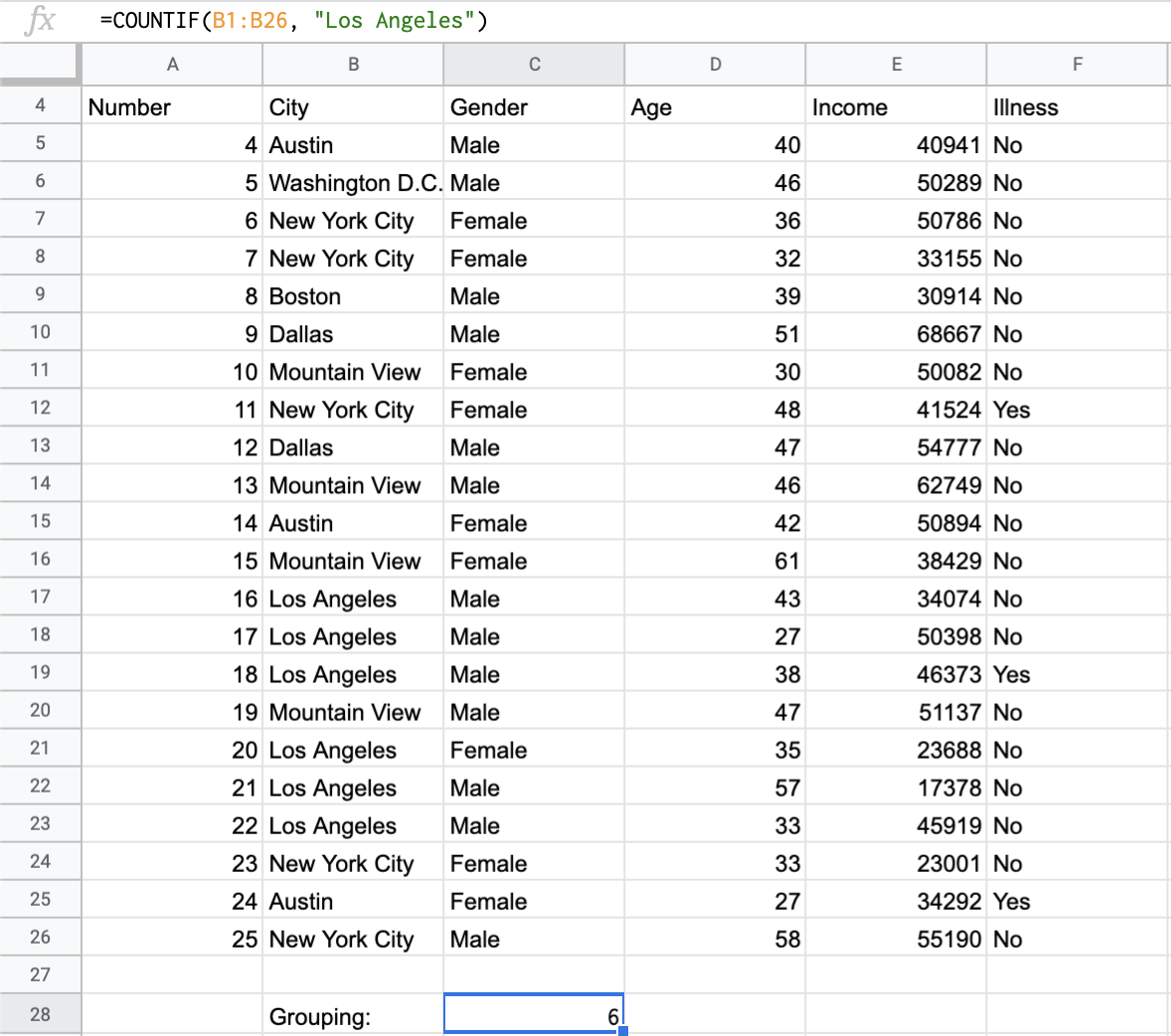The SUMIF function sums the values of cells that satisfy a condition. The syntax looks like =SUMIF(cell range to be filtered, filter condition, cell range to be summed).

Given the same table of standard information about people, you can find the total income of those from New York City. Consider the formula =SUMIF(B2:B26, "=New York City", E2:E26). This sums the values in the cells E2:E26, but only using rows for which the cell in that row in column B matches the word “New York City”. The cell range to be filtered is B2:B26, the filter condition is “=New York City”, and the cell range to be summed is E2:E26.

Pictured is a screenshot of part of the dataset in Sheets with the grouping function SUMIF.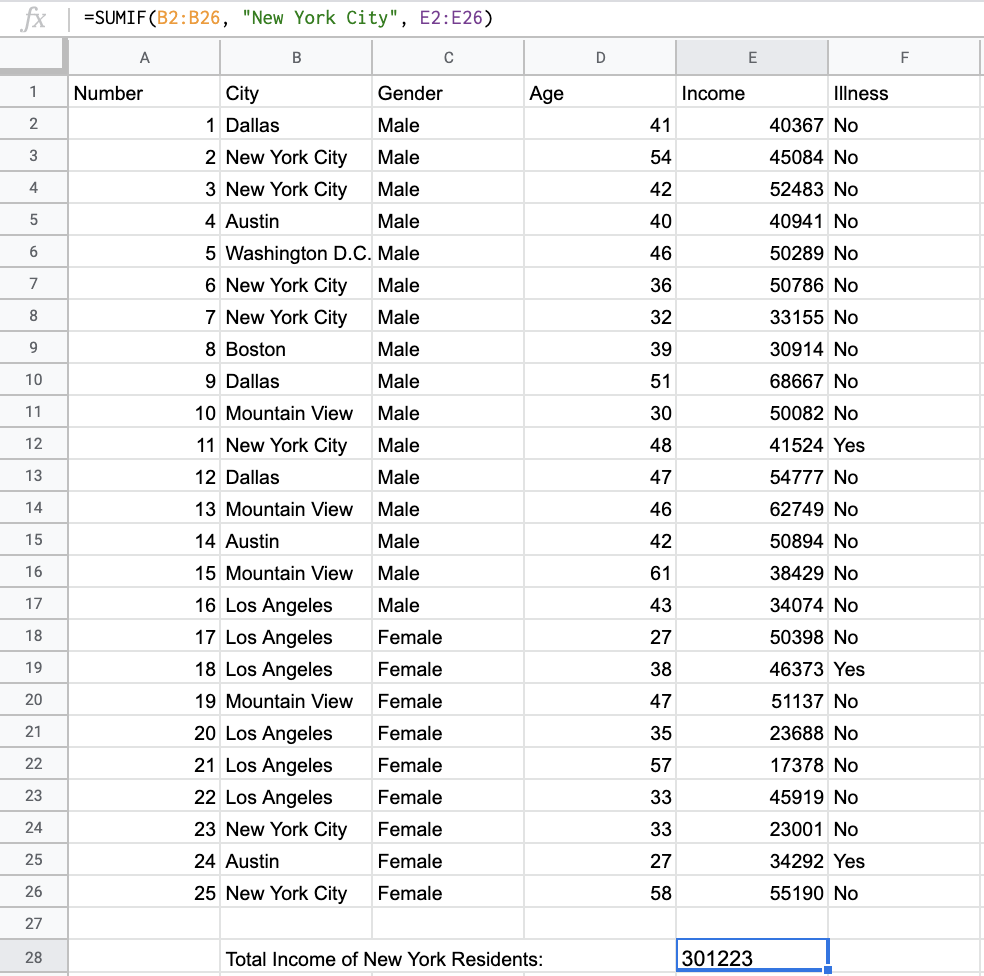The AVERAGEIF function finds the mean of cells that satisfy a condition. The syntax looks like =AVERAGEIF(cell range to be filtered, filter condition, cell range to be averaged).

Given a table of the top 50 songs on Spotify in 2019, you can calculate the mean popularity of songs by a certain artist. For example, consider the formula =AVERAGEIF(C4:C53, "=Ed Sheeran", E4:E53). This finds the mean of cells in the range E4:E53, but only using rows for which the cell in that row in column C matches the word “Ed Sheeran.” The cell range to be filtered is C4:C53, the filter condition is “=Ed Sheeran”, and the cell range to be averaged is E4:E53.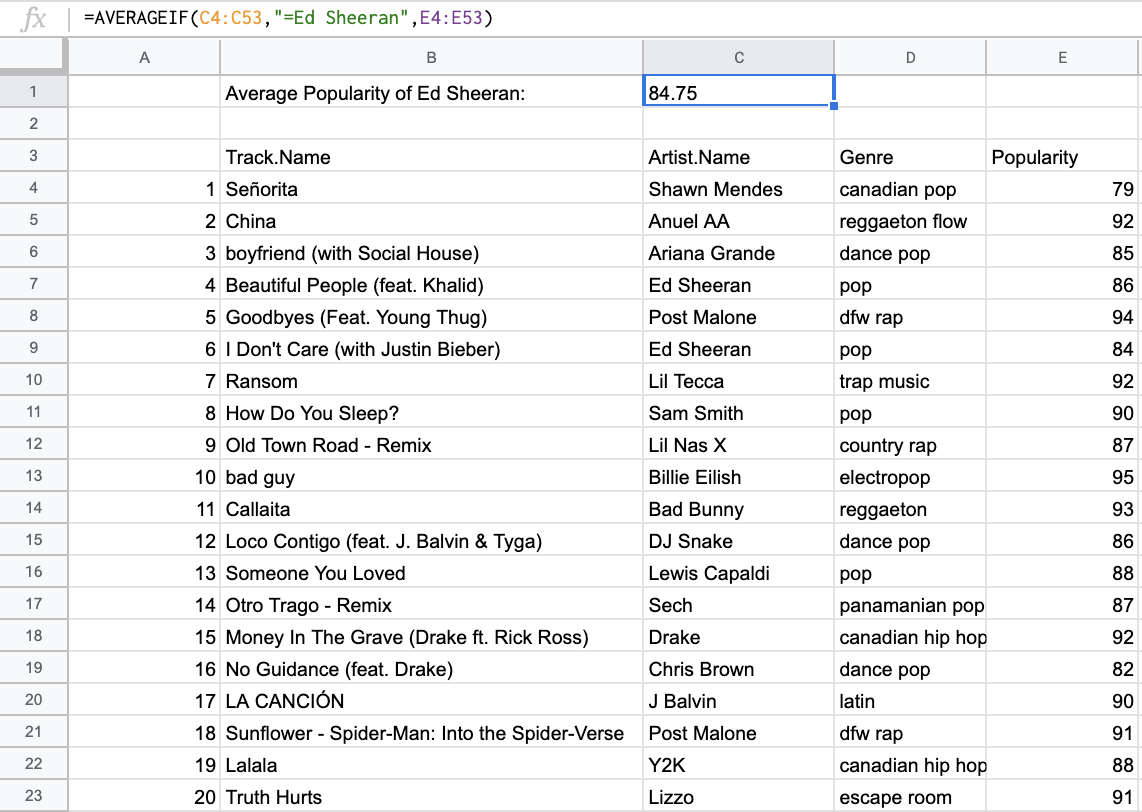Note that for SUMIF and AVERAGEIF, if you want to sum or average the rows in column B or C themselves, you can enter B2:B26 or C2:C53 as the third argument or leave the third argument empty.

## Example: Painters¶

Grouping functions are most useful when finding summary statistics for a specific group. Consider the painters dataset, one last time. Suppose you want to count the number of French painters in this dataset, and find the total number of paintings made by those painters. This can be done using filtering or using grouping.

Create a filter to limit the dataset to only French painters. (For this example, assume “French” means the only nationality is French.) Copy the filtered table to a new sheet.

Why was it necessary to copy-paste the filtered data to new rows? Cell ranges do not behave well in filtered tables. This is best illustrated via an example. Consider the filter applied above, to limit the painters dataset to French painters.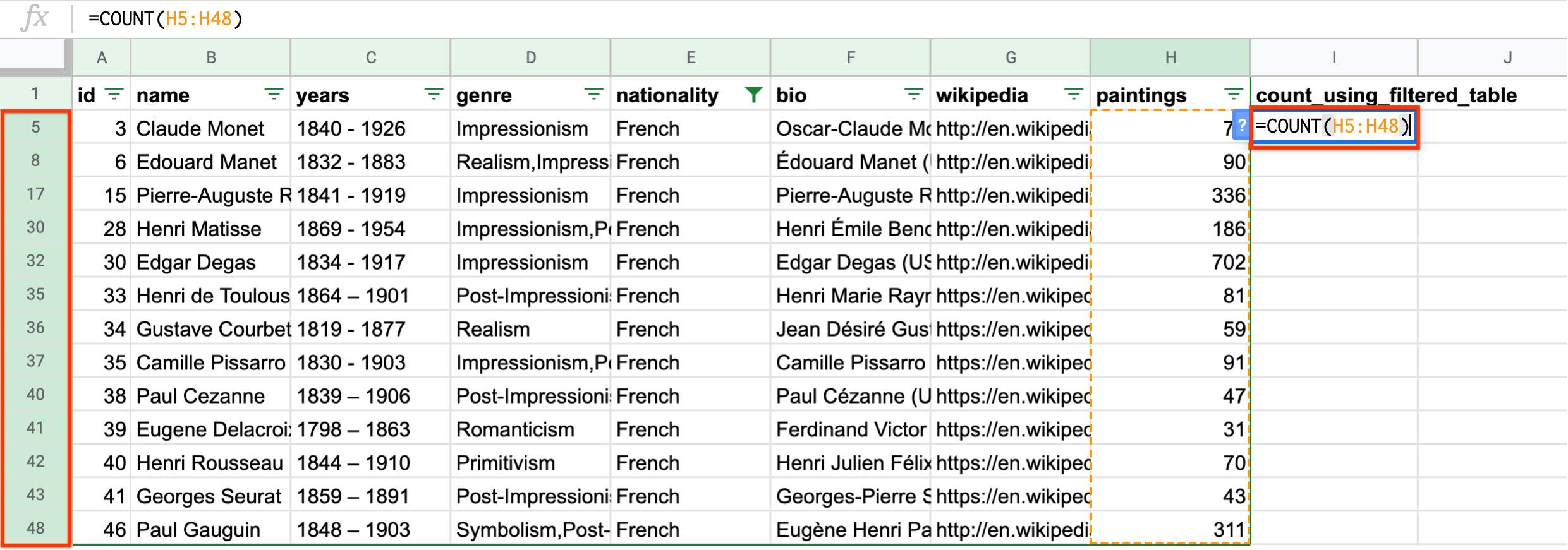Notice that when trying to count the number of rows, selecting the cells selects all cells between the first and the last, not just the filtered cells. Note that the selected range is H5:H48. While there are only 13 filtered rows, the range selects all rows between 5 and 48. This returns a count of 44 French artists, which is incorrect. The same is true for cell ranges when using SUM and AVERAGE.

Instead of copy-pasting the filtered table to a new sheet and then using summary functions, you can just use grouping functions. For example, to count the number of French painters, you can use COUNTIF. The range to be counted is the “nationality” column, and the column condition checks whether the value is “French”.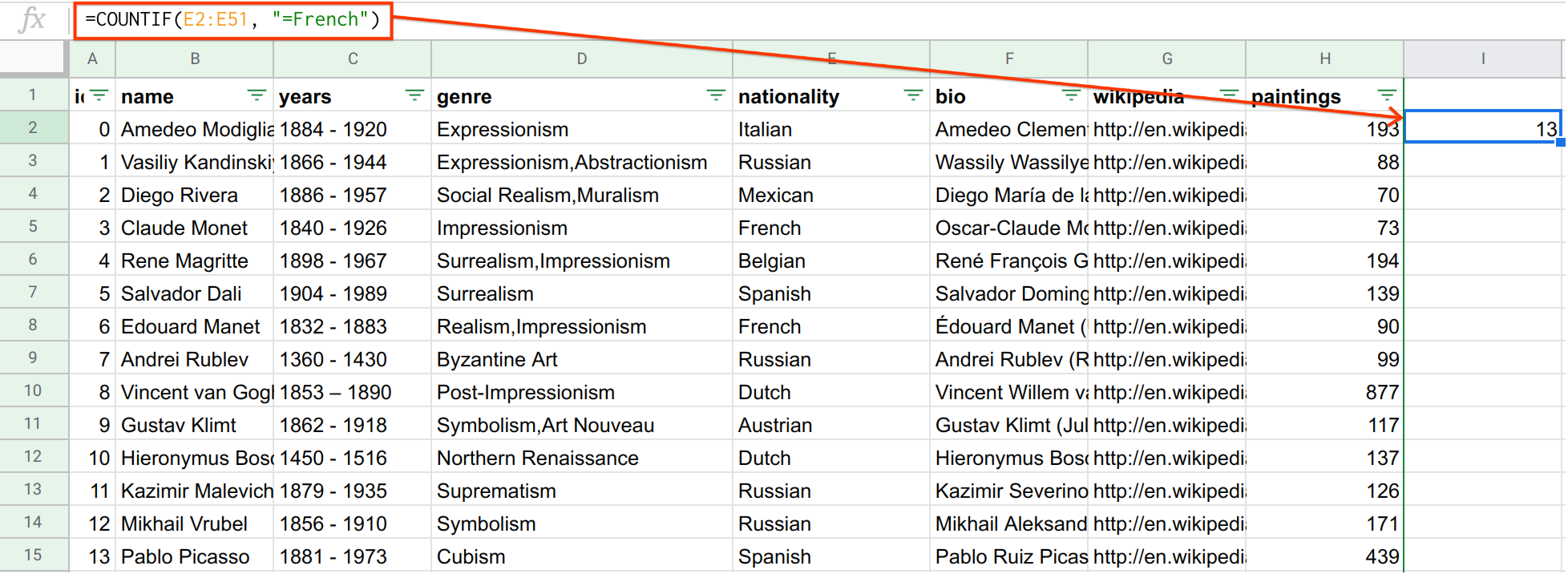Note that if you wanted to use the more general definition of “French” (any painter who has French as one of their nationalities), you would need to use a different filter condition. In general, the filter condition for checking if “word” appears anywhere in the text looks like "*word*". This forum discussion goes into more detail. This can apply to any grouping function.

Use grouping functions (COUNTIF, SUMIF, AVERAGEIF) when answering the following questions.

## Example: Titanic¶

The Titanic was a passenger ship that sank on its journey from Southampton (England) to New York (USA) in 1912, killing over 1,500 people. This example uses passenger data from the tragedy. Each row records a passenger on the ship.

The purpose of this example is to find out whether some groups, for example, women and children who had priority access to life rafts in case of emergency, had a higher survival rate than others. For example, did women and children have a higher survival rate than men? This can be done very conveniently using grouping functions.

In its raw state, the survival of each passenger is encoded as “Dead” or “Alive” in column B. These words are hard to deal with numerically, so you should probably first transform these values to numbers. For example, the following formula maps “Dead” to 0 and “Alive” to 1. (The example is for cell B2, but it can be copy-pasted for the other rows.) You can insert a column on the left of column C and use this column for the formula.

=IF(B2=“Alive”, 1, 0)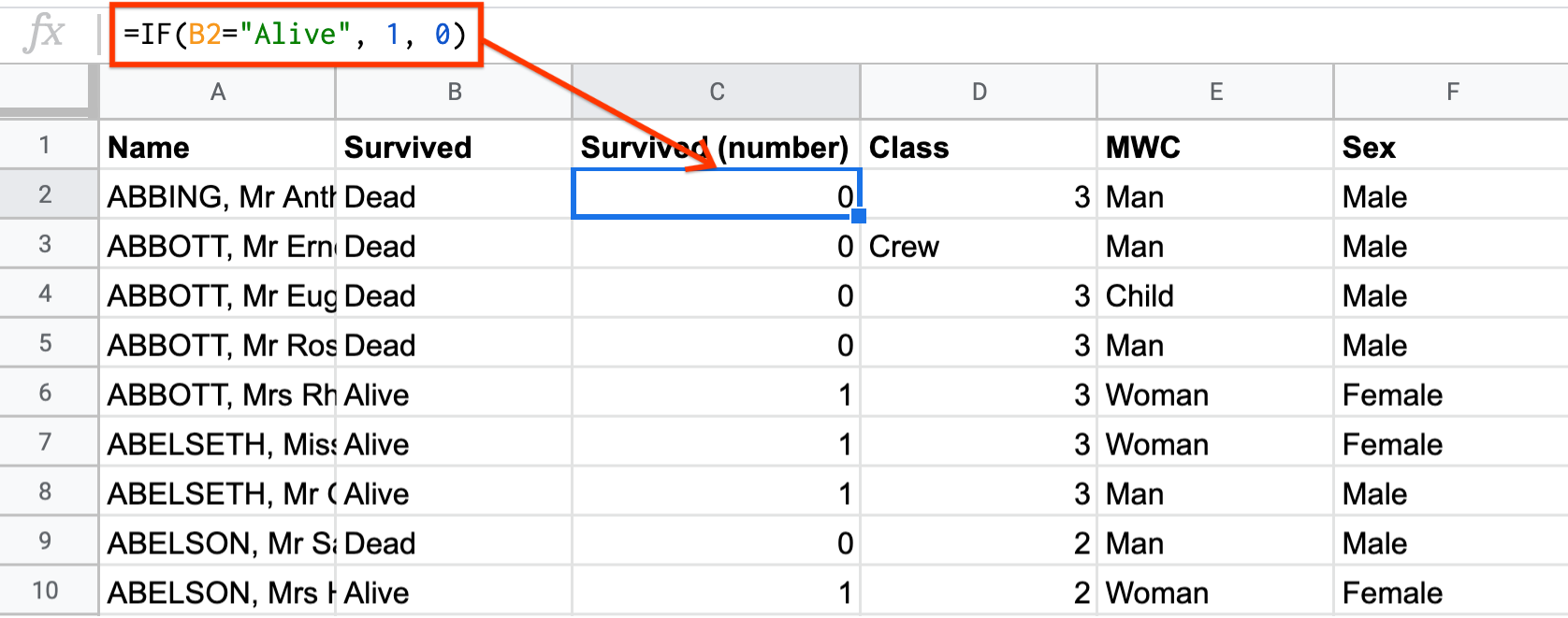This survival rate you just calculated is the overall survival rate for all passengers. What if you want to know the survival rate just for men, or just for women, or just for children?

To calculate the survival rate just for men, you need to find the mean of column C, but only if column E is equal to “Man”. This is a perfect use case for AVERAGEIF.

=AVERAGEIF(E$2:E$2209, "=Man", C$2:C$2209)
`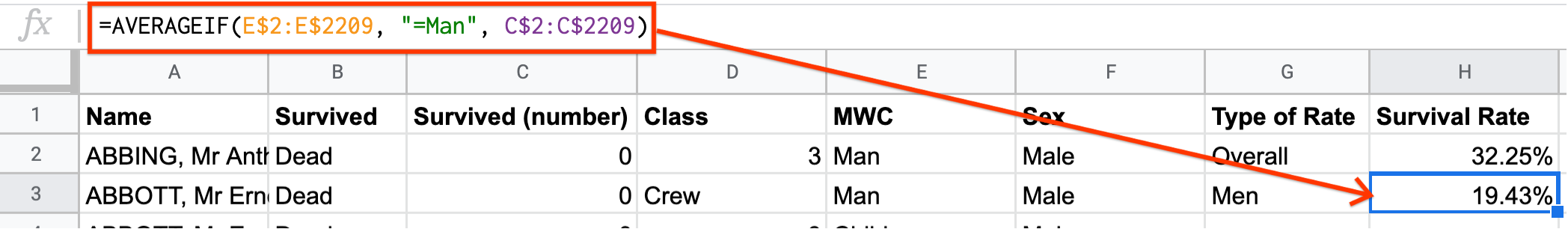Q-11: Compare the survival rate across the classes: Crew, Class 3, Class 2, Class 1. Is this in line with what you expected?